Function Repository Resource:

# ProjectOnSubspace

Project a vector onto a subspace

Contributed by: Dennis M Schneider
 ResourceFunction["ProjectOnSubspace"][u,v] finds the projection of the vector u onto the vector v. ResourceFunction["ProjectOnSubspace"][u,v,ip] finds the projection using the inner product ip. ResourceFunction["ProjectOnSubspace"][u,{v1,v2,…}] finds the projection of u onto the subspace spanned by {v1,v2,…}. ResourceFunction["ProjectOnSubspace"][u,{v1,v2,…},ip] finds the projection onto the subspace using the inner product ip.

## Examples

### Basic Examples (3)

The projection of one vector onto a 1D subspace:

 In:=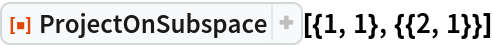Out=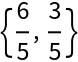This is the same as Mathematica’s Projection command, which projects one vector onto another:

 In:=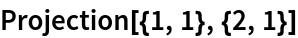Out=The projection of a vector onto a subspace spanned by a list of vectors:

 In:=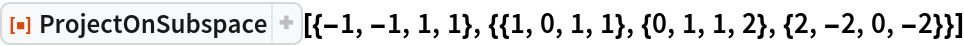Out=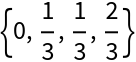The projection using a weighted inner product:

 In:=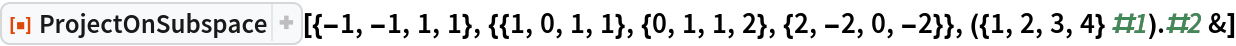Out=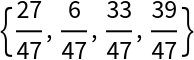### Scope (3)

Vectors with symbolic entries are assumed to represent complex numbers:

 In:=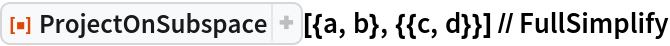Out=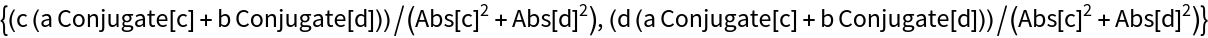By using Dot as the inner product, Mathematica will assume all symbolic entries are real:

 In:=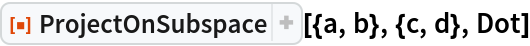Out=Project a vector in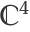onto a subspace of:

 In:=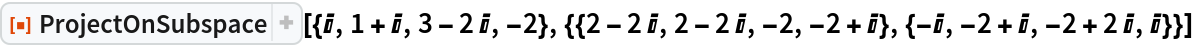Out=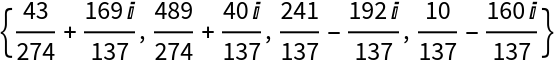Project a vector inonto a subspace ofhaving an orthogonal basis:

 In:=Out=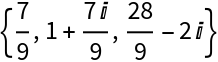Verify that the basis is orthonormal:

 In:=Out=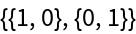Since the basis is orthonormal, the projection is the sum of the projections of the vector onto the orthonormal basis vectors:

 In:=Out=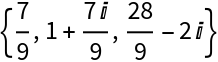#### Projections in spaces of matrices (2)

The projection of one matrix onto another using the standard matrix inner product:

 In:=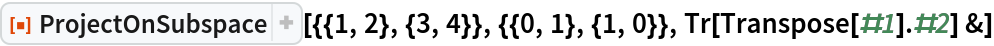Out=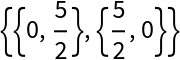The projection of a matrix onto a subspace of the space of 2×2 symmetric matrices using the standard matrix inner product:

 In:=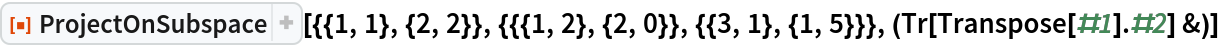Out=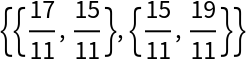#### Projections in function spaces (1)

The projection of x2 onto the subspace of trigonometric polynomials of degree 1 with the inner product Integrate[#1#2,{x,0,2π}]& gives the least-squares approximation to x2 by a function in the subspace of C[0,2π] spanned by 1,Sin[x],Cos[x]:

 In:=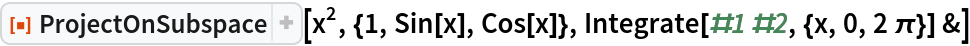Out=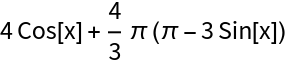## Publisher

Dennis M Schneider

## Version History

• 1.0.0 – 12 August 2019